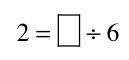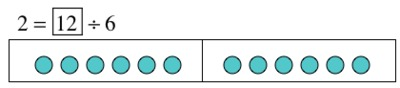Finding the unknown in a division equation

Alignments to Content Standards: 3.OA.A.4

Tehya and Kenneth are trying to figure out which number could be placed in the box to make this equation true.

Tehya insists that 12 is the only number that will make this equation true.

Kenneth insists that 3 is the only number that will make this equation true.Who is right? Why? Draw a picture to support your idea.

IM Commentary

This task shows an equation that elicits key misconceptions around equations and division. Students will be tempted to identify 3 as the missing number because they are envisioning the related fact:Teachers using this task for discussion will want students to voice ideas leading to both answers, but guide the discussion to some key instructional points:

• An equal sign indicates that both expressions have the same value, therefore 2 must have the same value as the mystery number divided by 6.
• Addition and multiplication are commutative, but division is not (so mentally plugging in 3 and dividing 3 by 6 does not give the same result as 6 divided by 3).
• Students may insist that the 2 belongs on the right side because it is "the answer." Since the equal sign shows that both sides have the same value, the equation can be written in either order (symmetric property of equality).
• There are different pictures that could be drawn to show that 12 is the correct answer. Students may split 12 into 6 groups of 2 or into 2 groups of 6.

Solution

This solution shows that 12 split into groups of 6 will result in 2 groups.This solution shows that 12 split into 6 equal groups will result in 2 in each group.